### Home > A2C > Chapter 9 > Lesson 9.1.3 > Problem9-62

9-62.
1. Without using a calculator, write the solution to each equation. Homework Help ✎

1. 2x = 17

2. log3(x + 1) = 5

3. log3(3x) = 4

4. 4log4(x) = 7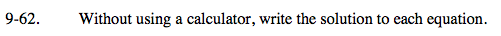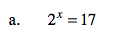Remember how to change the base using logarithms.$\text{\frac{log17}{log2}}$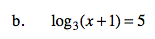242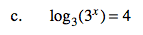4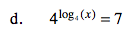7